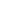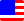Documents  >  Tutorial  >  group5
Operators of the 5th group

Purpose of this chapter.

We will learn about the relational operators.

Operators of the 5th group.

Operators of the 5th group are following.

 < x < y less than operator <= x <= y less than or equal operator > x > y greater than operator >= x >= y greater than equal operator

These operators are called relational operators. Relational operators compare the magnitude relationship of operands and return the result as a boolean value.

Relational operators compare magnitude relationships of operands, so operands must be numeric. That is, it must be one of the int, long, and real class. If you apply relational operators to other classes, an exception will be thrown.

Also, the classes of the left and right operands of relational operator must be the same. If the classes of the left and right operands are different, an exception will be thrown.(Changed in Ver 1.0.1.2)

The operands of relational operators may be different classes if they are numeric.

less than operator

The less than operator returns true if the left operand is less than the right operand. Otherwise, it returns false.

For example,

bool result0 = 10 < 11; //The result is true;

bool result1 = 10 < 10; //The result is false;

bool result2 = 11 < 10; //The result is false;

less than or equal operator

The less than or equal operator returns true if the left operand is less than or equal to the right operand. Otherwise, it returns false.

For example,

bool result0 = 10 <= 11; //The result is true;

bool result1 = 10 <= 10; //The result is true;

bool result2 = 11 <= 10; //The result is false;

greater than operator

The greater than operator returns true if the left operand is greater than the right operand. Otherwise, it returns false.

For example,

bool result0 = 10 > 11; //The result is false;

bool result1 = 10 > 10; //The result is false;

bool result2 = 11 > 10; //The result is true;

greater than or equal operator

The greater than or equal operator returns true if the left operand is greater than or equal to the right operand. Otherwise, it returns false.

For example,

bool result0 = 10 >= 11; //The result is false;

bool result1 = 10 >= 10; //The result is true;

bool result2 = 11 >= 10; //The result is true;